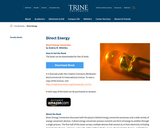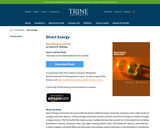Updating search results...

# 2 Results

View
Selected filters:
• Trine UniversityConditional Remix & Share Permitted
CC BY-NC
Rating
0.0 stars

Direct Energy Conversion discusses both the physics behind energy conversion processes and a wide variety of energy conversion devices. A direct energy conversion process converts one form of energy to another through a single process. The first half of this book surveys multiple devices that convert to or from electricity including piezoelectric devices, antennas, solar cells, light emitting diodes, lasers, thermoelectric devices, and batteries. In these chapters, physical effects are discussed, terminology used by engineers in the discipline is introduced, and insights into material selection is studied. The second part of this book puts concepts of energy conversion in a more abstract framework. These chapters introduce the idea of calculus of variations and illuminate relationships between energy conversion processes.

Subject:
Applied Science
Engineering
Material Type:
Textbook
Provider:
Trine University
Author:
Andrea Mitofsky
01/01/2018Conditional Remix & Share Permitted
CC BY-NC
Rating
0.0 stars

Direct Energy Conversion discusses both the physics behind energy conversion processes and a wide variety of energy conversion devices. A direct energy conversion process converts one form of energy to another through
a single process. The first half of this book surveys multiple devices that convert to or from electricity including piezoelectric devices, antennas, solar cells, light emitting diodes, lasers, thermoelectric devices, and batteries.
In these chapters, physical effects are discussed, terminology used by engineers in the discipline is introduced, and insights into material selection is studied. The second part of this book puts concepts of energy conversion
in a more abstract framework. These chapters introduce the idea of calculus of variations and illuminate relationships between energy conversion processes.

This peer-reviewed book is used for a junior level electrical engineering class at Trine University. However, it is intended not just for electrical engineers. Direct energy conversion is a fascinating topic because it does not fit neatly into a single discipline. This book also should be of interest to physicists, chemists, mechanical engineers, and other researchers interestedin an introduction to the energy conversion devices studied by scientists and engineers in other disciplines

Subject:
Applied Science
Engineering
Material Type:
Textbook
Provider:
Trine University
Author:
Andrea Mitofsky